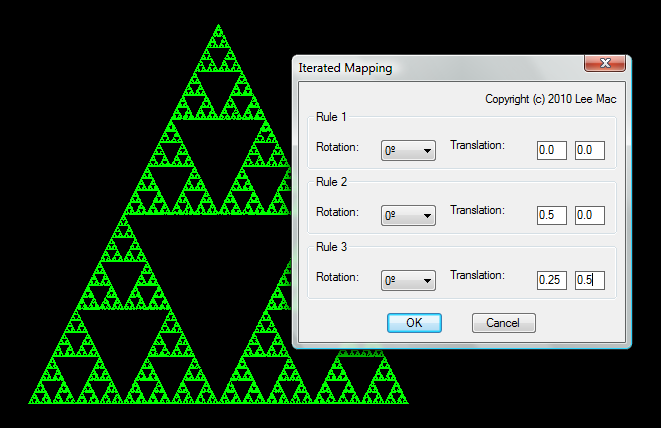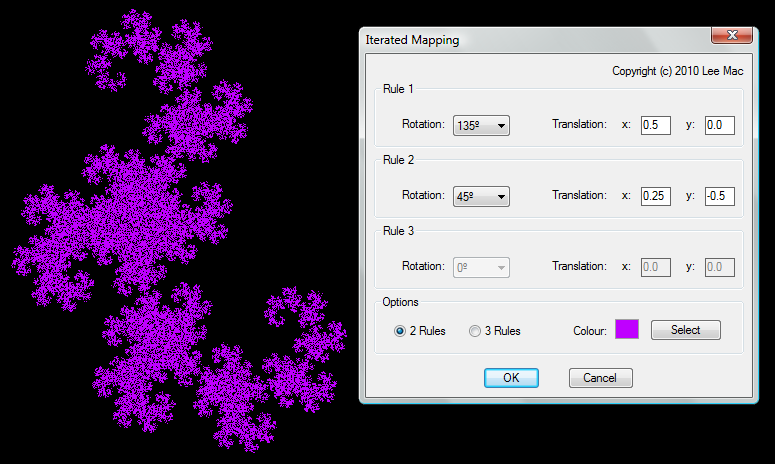Iterated Function Systems

 Function Syntax IFS Current Version 1.2 Download IteratedFunctionSystemsV1-2.lsp View HTML Version IteratedFunctionSystemsV1-2.html

I really enjoyed writing this one, not only due to the mathematics involved, but the elegance in that such a complex graphic may be encoded using a few simple rules.

Program Description

I started by modelling the famous Barnsley's Fern, an example of an Iterated Function System (IFS) in which a function is applied recursively to generate further points in the set.

Such functions are contraction mappings meaning points are always mapped closer together, hence, by its recursive nature, a fractal is consequently generated. In this case, I use an affine linear function, operating with various matrices & vectors weighted with specific probabilities to produce the desired result.

The mapping takes the form:In which A is a matrix, and b is a vector, in functional notation, this may be seen as:Barnsley's Fern

The following code takes an initial point and applies a map to it, plots the result, then recursively applies a map to the result, the matrix & vector combination used in the map depend upon a generated probability.

Select all
;;-------------------=={ Barnsley's Fern }==------------------;;
;;                                                            ;;
;;  Function uses an iterated map to recursively calculate    ;;
;;  point positions, creating the image of Barnsley's Fern.   ;;
;;------------------------------------------------------------;;
;;------------------------------------------------------------;;

(defun c:fern ( / m p r v )
(setvar 'pdmode 0)
(repeat 15000
(setq r (LM:rand))
(cond
(   (<       r 0.01) (setq m '(( 0.00  0.00) ( 0.00  0.16)) v '(0.00 0.00)))
(   (<= 0.01 r 0.86) (setq m '(( 0.85  0.04) (-0.04  0.85)) v '(0.00 1.60)))
(   (<  0.86 r 0.93) (setq m '(( 0.20 -0.26) ( 0.23  0.22)) v '(0.00 1.60)))
(                    (setq m '((-0.15  0.28) ( 0.26  0.24)) v '(0.00 0.44)))
)
(entmake (list '(0 . "POINT") '(62 . 3) (cons 10 (setq p (mapcar '+ (mxv m p) v)))))
)
(princ)
)

;; Rand  -  Lee Mac
;; PRNG implementing a linear congruential generator with
;; parameters derived from the book 'Numerical Recipes'

(defun LM:rand ( / a c m )
(setq m   4294967296.0
a   1664525.0
c   1013904223.0
\$xn (rem (+ c (* a (cond (\$xn) ((getvar 'date))))) m)
)
(/ \$xn m)
)

;; Matrix x Vector  -  Vladimir Nesterovsky
;; Args: m - nxn matrix, v - vector in R^n

(defun mxv ( m v )
(mapcar '(lambda ( r ) (apply '+ (mapcar '* r v))) m)
)

ResultAn Extension to Generality

The above code uses a predetermined set of matrices & vectors to create the desired graphic (of a fern), so I decided to pursue this idea further, to create a more general program so that a user may choose the rules by which the image is generated.

The program available for download offers this functionality. The user may choose to apply two or three rules, each with a rotation and/or a translation. In this way, one can generate many famous figures, such as Sierpinski's Triangle, or a De Rham Curve.

Some Examples